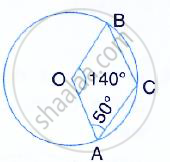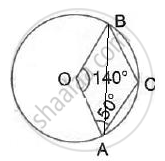Share

# In the Given Figure, O is the Centre of the Circle. If ∠Aob = 140° and ∠Oac = 50°; Find: (I) ∠Acb, (Ii) ∠Obc, (Iii) ∠Oab, (Iv) ∠Cba. - Mathematics

Course

#### Question

In the given figure, O is the centre of the circle. If ∠AOB = 140° and ∠OAC = 50°; Find:
(i) ∠ACB,  (ii) ∠OBC,  (iii) ∠OAB,  (iv) ∠CBA.#### SolutionHere, ∠ACB = 1/2Reflex (∠AOB) =1/2 (360° -140°) = 110°
(Angle at the centre is double the angle at the circumference subtended by the same chord)
Now, OA = OB (Radii of same circle)
∴ ∠OBA = ∠OAB = (180° -140°)/2= 20°
∴  ∠CAB = 50° - 20°= 30°
ΔCAB,
∠CBA - 180° -110°- 30° = 40°
∴  ∠OBC = ∠CBA + ∠OBA = 40° + 20° = 60°

Is there an error in this question or solution?